# FLOW OF CONTROL Flow of Control Sequential flow

• Slides: 28FLOW OF CONTROL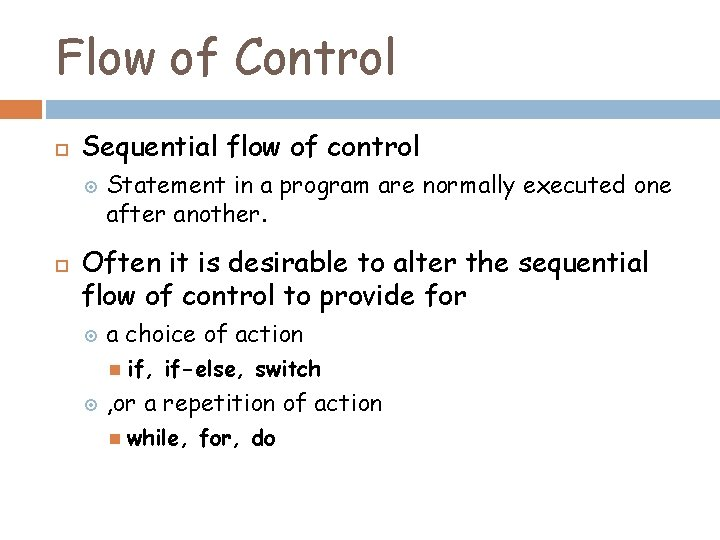Flow of Control Sequential flow of control Statement in a program are normally executed one after another. Often it is desirable to alter the sequential flow of control to provide for a choice of action if, if-else, switch , or a repetition of action while, for, do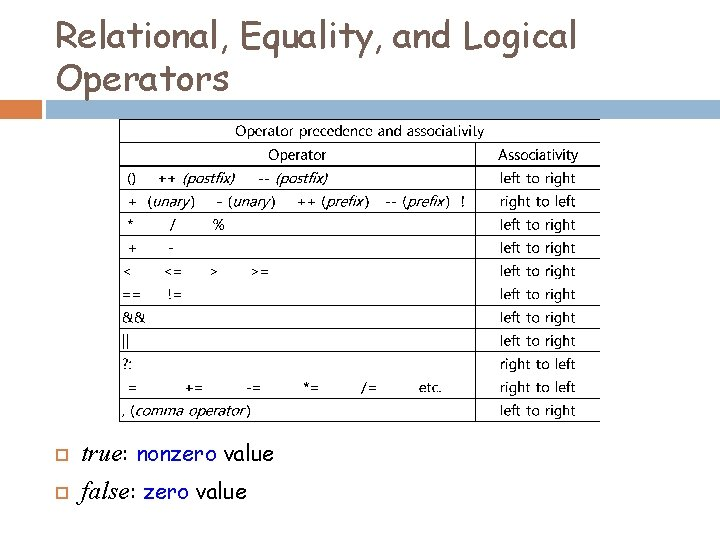Relational, Equality, and Logical Operators true: nonzero value false: zero valueRelational Operators and Expressions expr < expr > expr <= expr >= expr <Examples> a < 3 a =< b /* out of order */ a > b a < = b /* space not allowed*/ -1. 3 >= (2. 0 * x + 3. 3) <NOT Examples> a >> b /* shift expression */ a < b If a is less than b, then the expr. has the int value 1 (true). If a is not less than b, then the expr. has the int value 0 (false).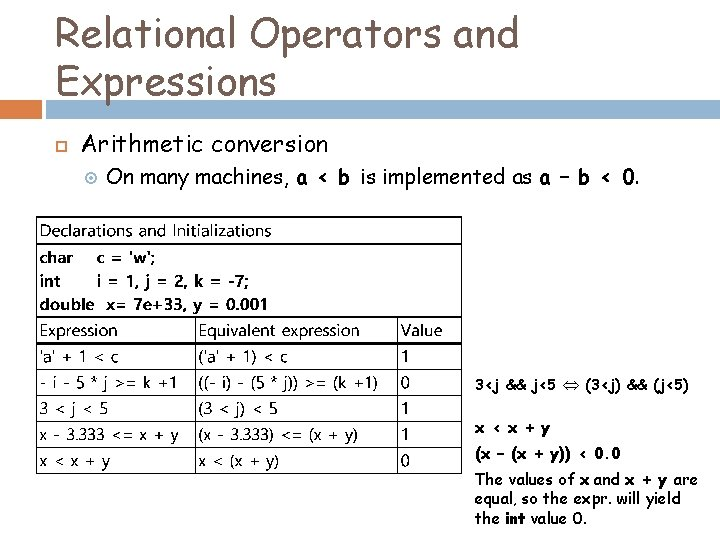Relational Operators and Expressions Arithmetic conversion On many machines, a < b is implemented as a – b < 0. 3<j && j<5 (3<j) && (j<5) x < x + y (x – (x + y)) < 0. 0 The values of x and x + y are equal, so the expr. will yield the int value 0.Equality Operators and Expressions expr == expr != expr <Examples> <NOT Examples> c == ‘A’ a = b /* assignment */ k != -2 a = = b - 1 /* space not allowed*/ x + y == 3 * z – 7 (x + y) =! 44 /* (x + y) = (!44) */ a == b is either true or false is implemented as a – b == 0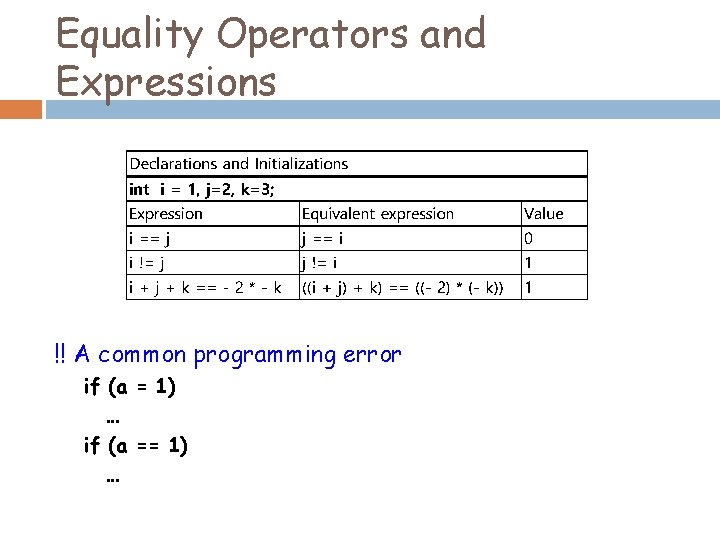Equality Operators and Expressions !! A common programming error if (a = 1) … if (a == 1) …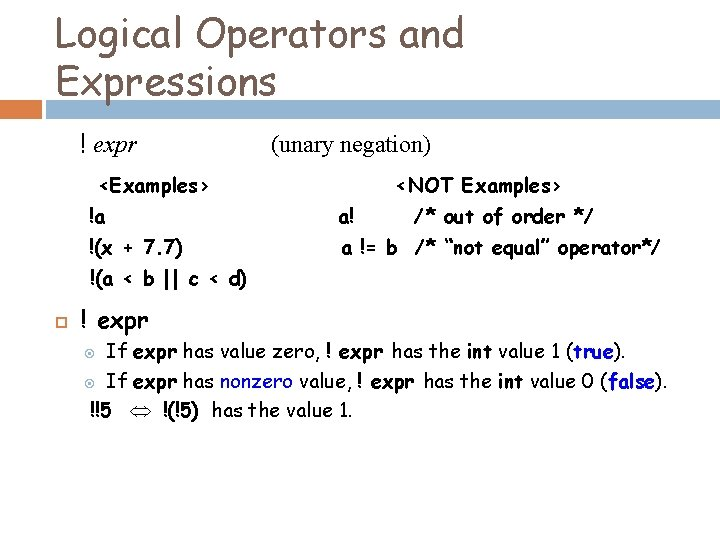Logical Operators and Expressions ! expr (unary negation) <Examples> <NOT Examples> !a a! /* out of order */ !(x + 7. 7) a != b /* “not equal” operator*/ !(a < b || c < d) ! expr If expr has value zero, ! expr has the int value 1 (true). If expr has nonzero value, ! expr has the int value 0 (false). !!5 !(!5) has the value 1.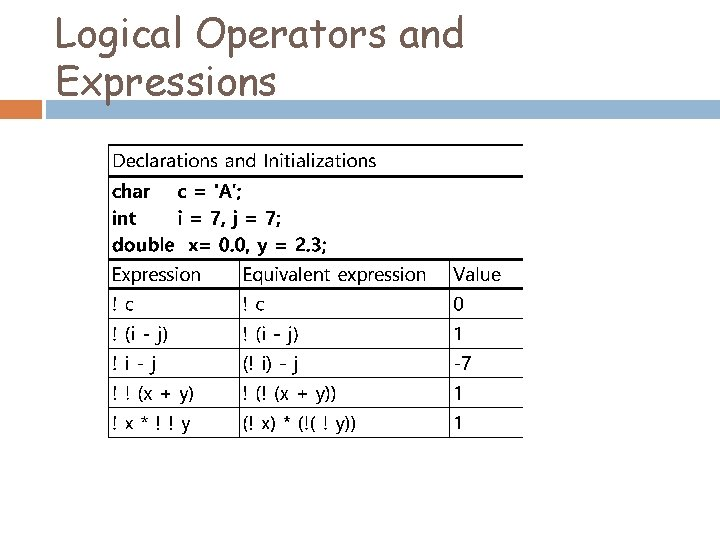Logical Operators and Expressions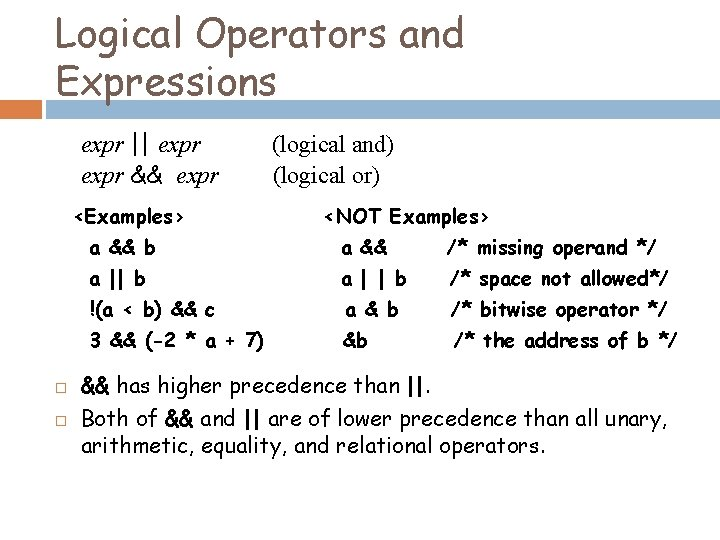Logical Operators and Expressions expr || expr && expr <Examples> (logical and) (logical or) <NOT Examples> a && b a && /* missing operand */ a || b a | | b /* space not allowed*/ !(a < b) && c a & b /* bitwise operator */ 3 && (-2 * a + 7) &b /* the address of b */ && has higher precedence than ||. Both of && and || are of lower precedence than all unary, arithmetic, equality, and relational operators.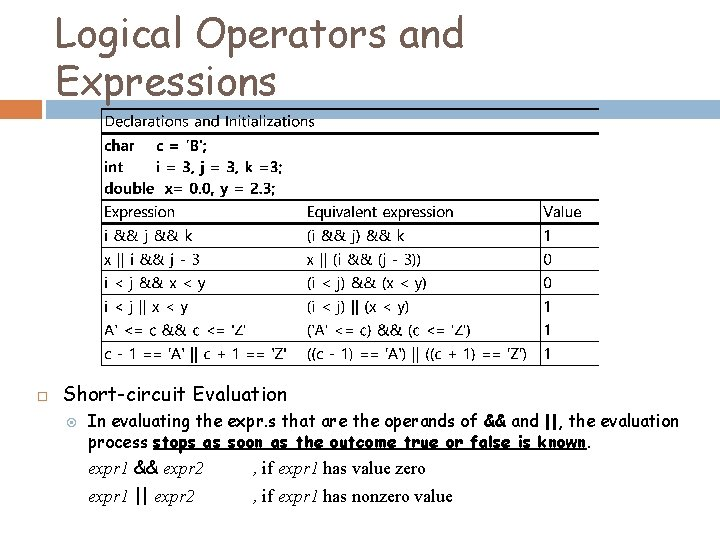Logical Operators and Expressions Short-circuit Evaluation In evaluating the expr. s that are the operands of && and ||, the evaluation process stops as soon as the outcome true or false is known. expr 1 && expr 2 , if expr 1 has value zero expr 1 || expr 2 , if expr 1 has nonzero value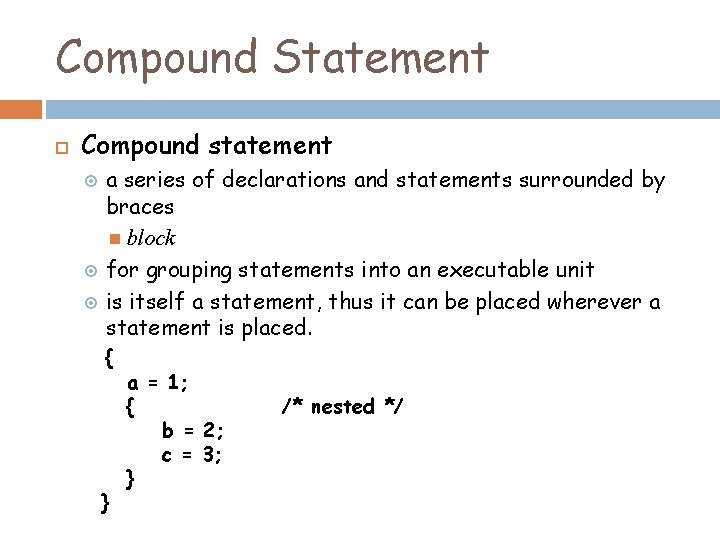Compound Statement Compound statement a series of declarations and statements surrounded by braces block for grouping statements into an executable unit is itself a statement, thus it can be placed wherever a statement is placed. { } a = 1; { b = 2; c = 3; } /* nested */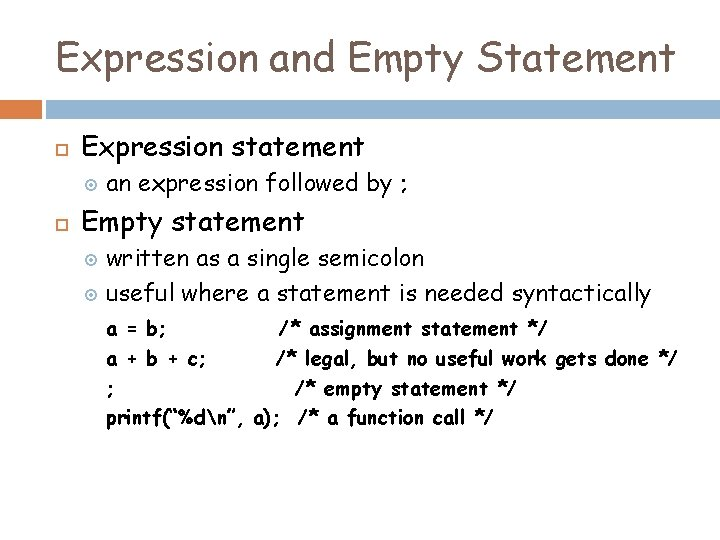Expression and Empty Statement Expression statement an expression followed by ; Empty statement written as a single semicolon useful where a statement is needed syntactically a = b; /* assignment statement */ a + b + c; /* legal, but no useful work gets done */ ; /* empty statement */ printf(“%dn”, a); /* a function call */if and if-else Statements if (expr) statement If expr is nonzero, then statement is executed; otherwise, statement is skipped and control passes to the next statement. if (j < k) { min = j; printf(“j is smaller than kn”); }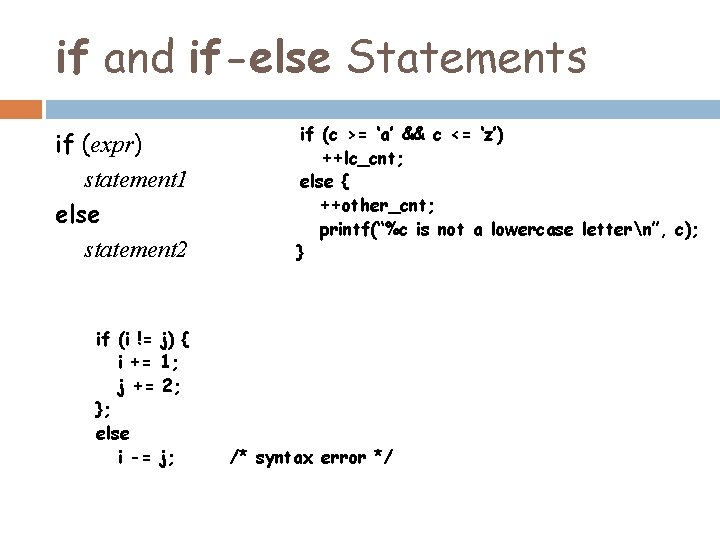if and if-else Statements if (expr) statement 1 else statement 2 if (i != i += j += }; else i -= if (c >= ‘a’ && c <= ‘z’) ++lc_cnt; else { ++other_cnt; printf(“%c is not a lowercase lettern”, c); } j) { 1; 2; j; /* syntax error */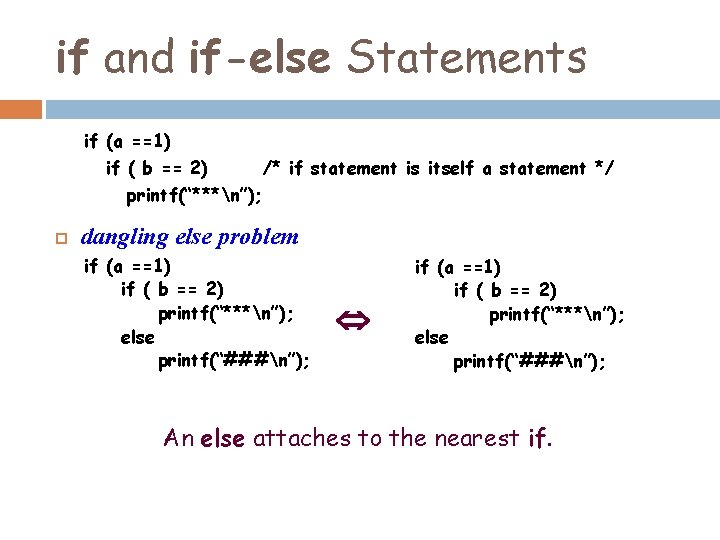if and if-else Statements if (a ==1) if ( b == 2) /* if statement is itself a statement */ printf(“***n”); dangling else problem if (a ==1) if ( b == 2) printf(“***n”); else printf(“###n”); An else attaches to the nearest if.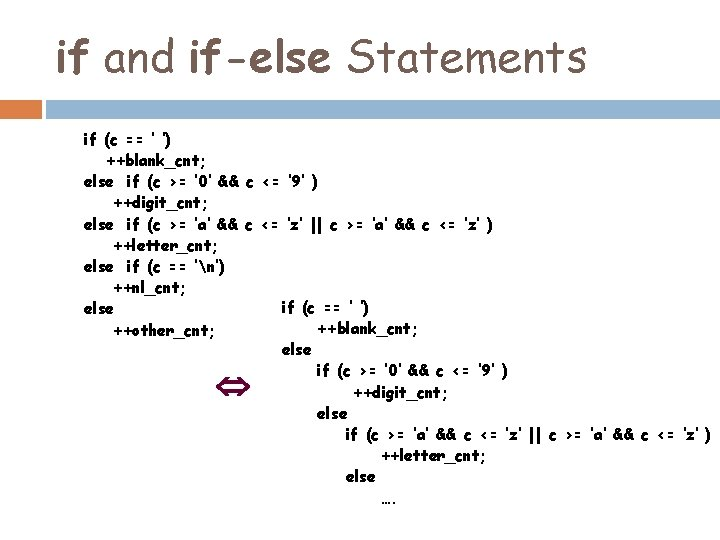if and if-else Statements if (c == ‘ ‘) ++blank_cnt; else if (c >= ‘ 0’ && c <= ‘ 9’ ) ++digit_cnt; else if (c >= ‘a’ && c <= ‘z’ || c >= ‘a’ && c <= ‘z’ ) ++letter_cnt; else if (c == ‘n’) ++nl_cnt; if (c == ‘ ‘) else ++blank_cnt; ++other_cnt; else if (c >= ‘ 0’ && c <= ‘ 9’ ) ++digit_cnt; else if (c >= ‘a’ && c <= ‘z’ || c >= ‘a’ && c <= ‘z’ ) ++letter_cnt; else ….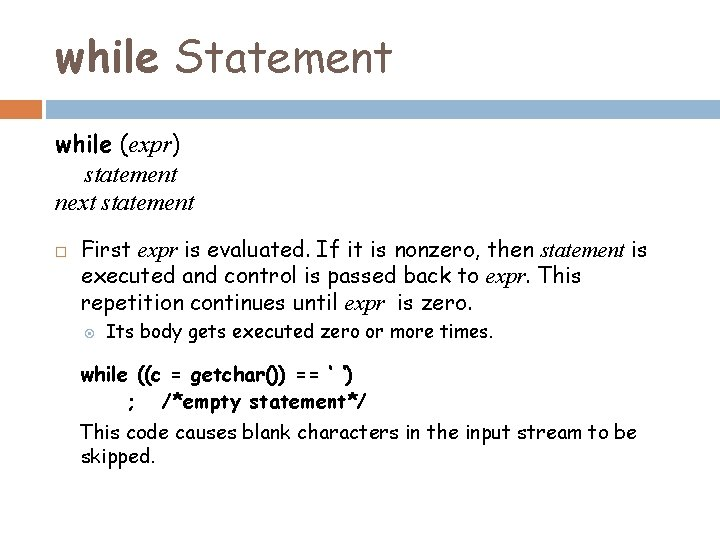while Statement while (expr) statement next statement First expr is evaluated. If it is nonzero, then statement is executed and control is passed back to expr. This repetition continues until expr is zero. Its body gets executed zero or more times. while ((c = getchar()) == ‘ ‘) ; /*empty statement*/ This code causes blank characters in the input stream to be skipped.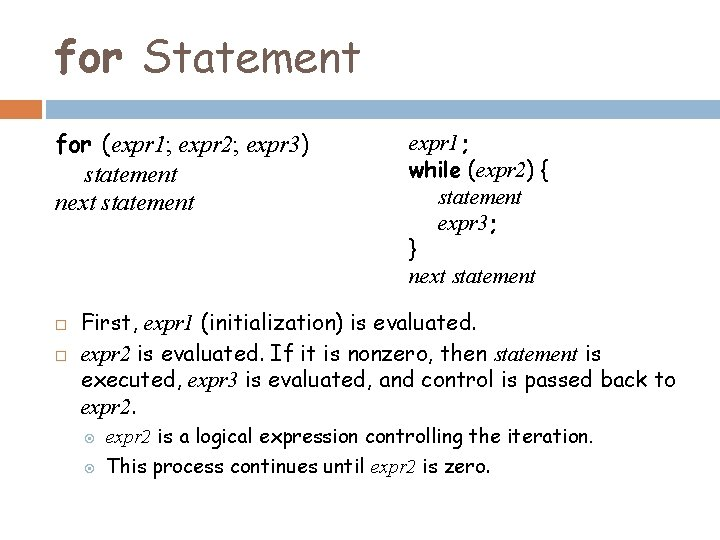for Statement for (expr 1; expr 2; expr 3) statement next statement expr 1; while (expr 2) { statement expr 3; } next statement First, expr 1 (initialization) is evaluated. expr 2 is evaluated. If it is nonzero, then statement is executed, expr 3 is evaluated, and control is passed back to expr 2 is a logical expression controlling the iteration. This process continues until expr 2 is zero.for Statement for (i =1; i <= n; ++i) factorial *= i; sum = 0; for (i =1; i <= 10; ++i) sum += i; sum = 0; i = 1; for ( ; ; ) sum += i++; Infinite Loop !! sum = 0; i = 1; for ( ; i <= 10; ++i) sum += i; sum = 0; i = 1; for ( ; i <= 10; ) sum += i++; for ( ………. . ) statement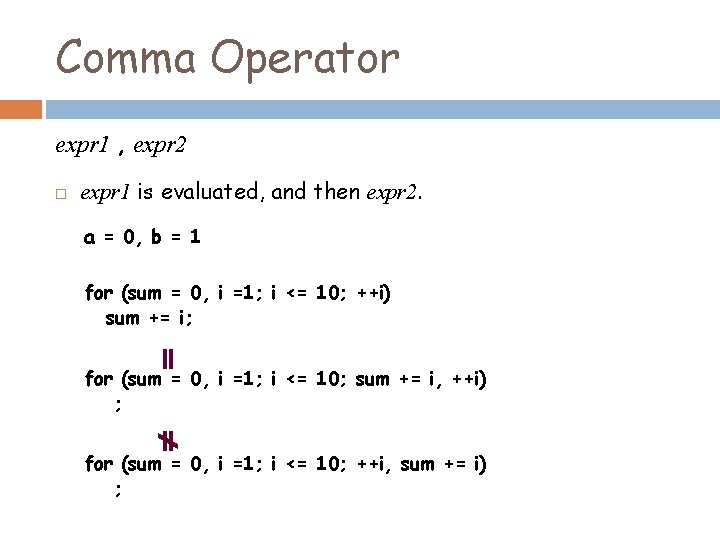Comma Operator expr 1 , expr 2 expr 1 is evaluated, and then expr 2. a = 0, b = 1 for (sum = 0, i =1; i <= 10; ++i) sum += i; for (sum = 0, i =1; i <= 10; sum += i, ++i) ; for (sum = 0, i =1; i <= 10; ++i, sum += i) ;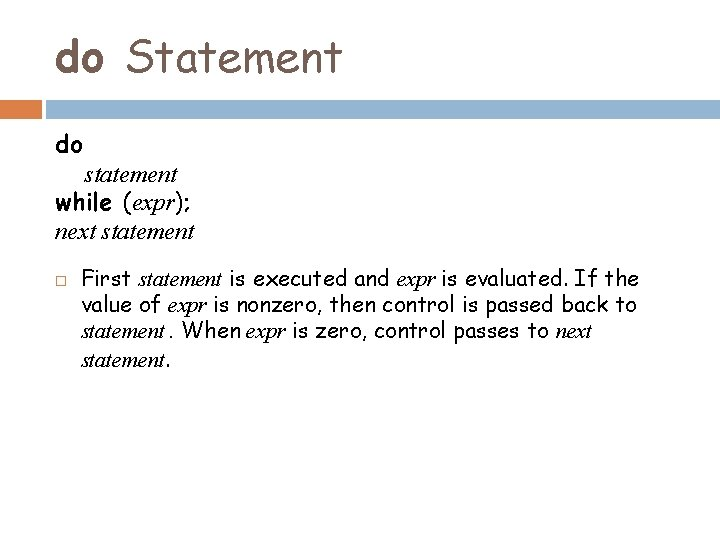do Statement do statement while (expr); next statement First statement is executed and expr is evaluated. If the value of expr is nonzero, then control is passed back to statement. When expr is zero, control passes to next statement.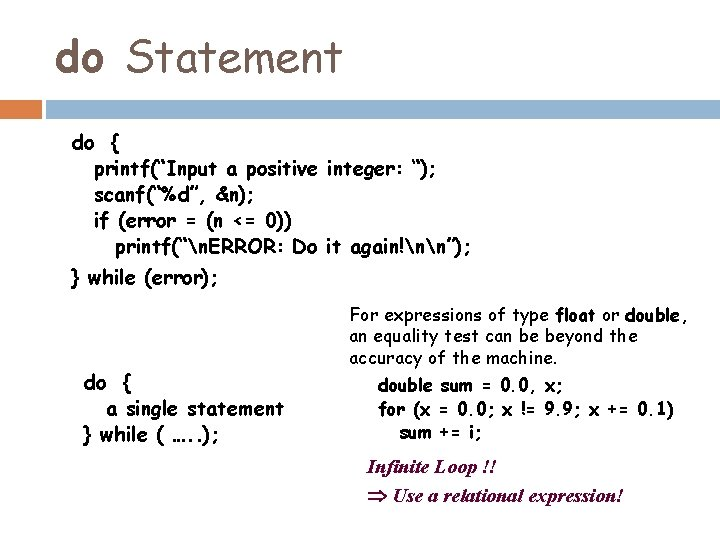do Statement do { printf(“Input a positive integer: “); scanf(“%d”, &n); if (error = (n <= 0)) printf(“n. ERROR: Do it again!nn”); } while (error); do { a single statement } while ( …. . ); For expressions of type float or double, an equality test can be beyond the accuracy of the machine. double sum = 0. 0, x; for (x = 0. 0; x != 9. 9; x += 0. 1) sum += i; Infinite Loop !! Use a relational expression!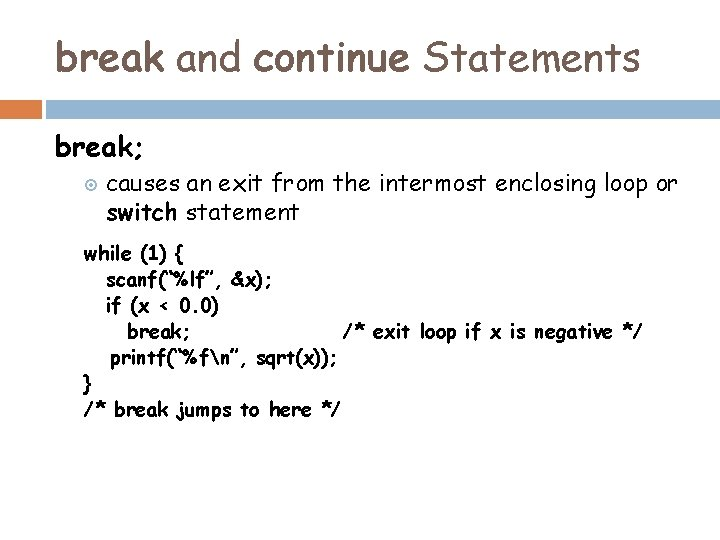break and continue Statements break; causes an exit from the intermost enclosing loop or switch statement while (1) { scanf(“%lf”, &x); if (x < 0. 0) break; /* exit loop if x is negative */ printf(“%fn”, sqrt(x)); } /* break jumps to here */break and continue Statements continue; causes the current iteration of a loop to stop and causes the next iteration of the loop to begin immediately for (i=0; i<TOTAL; ++i) { c = getchar(); if (c >= ‘ 0’ && c <= ‘ 9’) continue; …. . /* process other characters */ /*continue transfers control to here to begin next iteration*/ }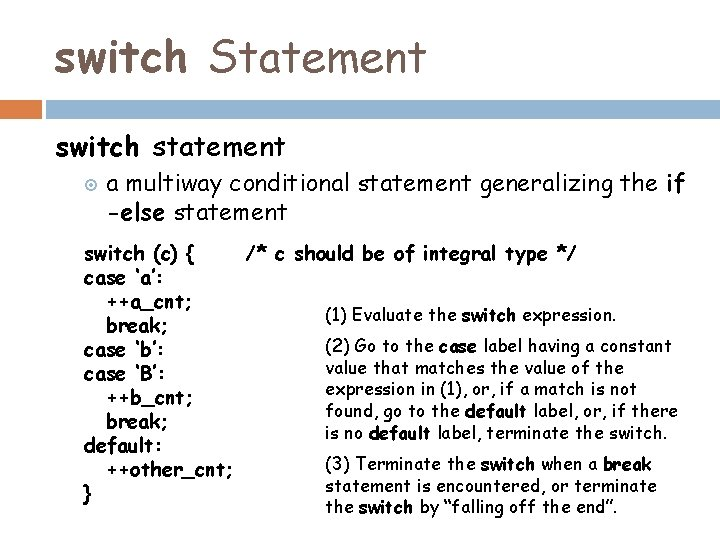switch Statement switch statement a multiway conditional statement generalizing the if -else statement switch (c) { /* c should be of integral type */ case ‘a’: ++a_cnt; (1) Evaluate the switch expression. break; (2) Go to the case label having a constant case ‘b’: value that matches the value of the case ‘B’: expression in (1), or, if a match is not ++b_cnt; found, go to the default label, or, if there break; is no default label, terminate the switch. default: (3) Terminate the switch when a break ++other_cnt; statement is encountered, or terminate } the switch by “falling off the end”.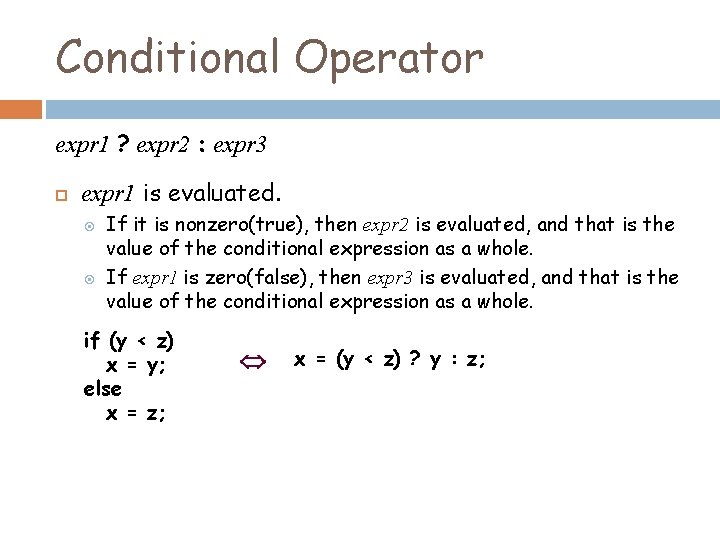Conditional Operator expr 1 ? expr 2 : expr 3 expr 1 is evaluated. If it is nonzero(true), then expr 2 is evaluated, and that is the value of the conditional expression as a whole. If expr 1 is zero(false), then expr 3 is evaluated, and that is the value of the conditional expression as a whole. if (y < z) x = y; else x = z; x = (y < z) ? y : z;Conditional Operator expr 1 ? expr 2 : expr 3 Its type is determined by both expr 2 and expr 3 Different types Usual Conversion Rules does not depend on which of expr 2 or expr 3 is evaluated.